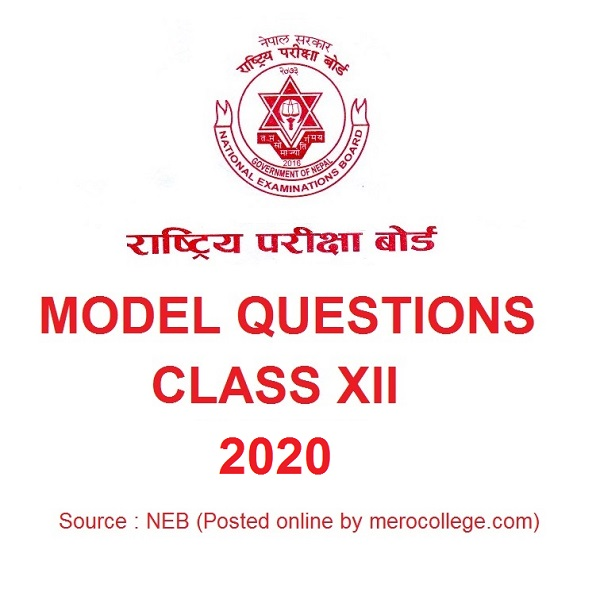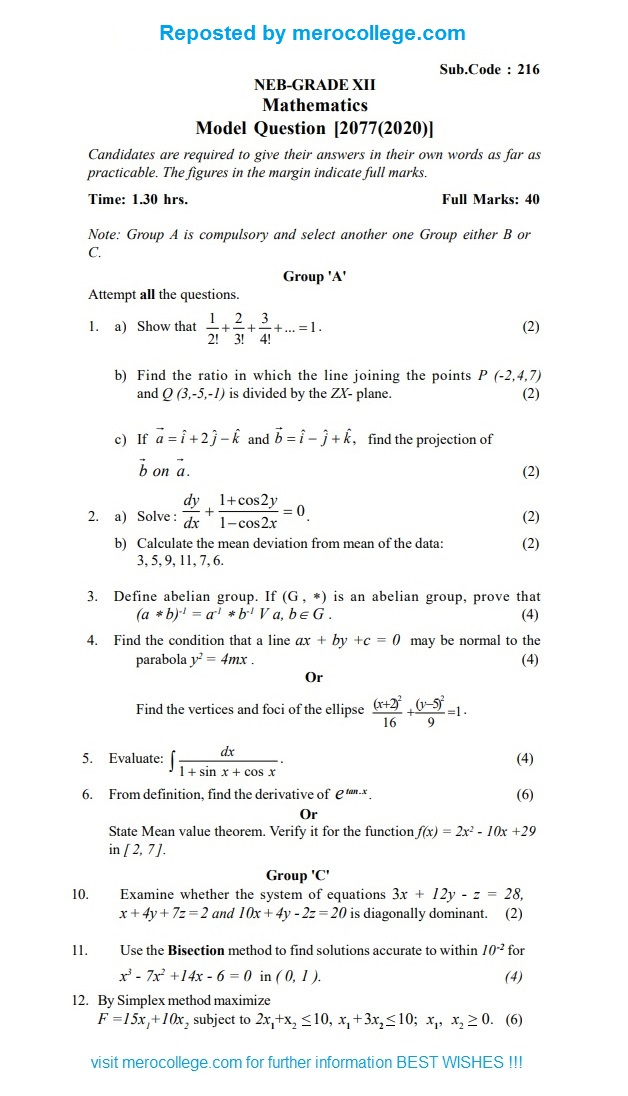# NEB 12 Model Questions 2020 Maths# NEB XII Model Questions 2020 Maths

NEB 12 Model Questions 2020 for Maths has been provided by National Examination Board, Nepal (NEB) for it’s upcoming Class XII Examinations.## NEB-GRADE XII Mathematics Model Question [2077(2020)]

Candidates are required to give their answers in their own words as far as practicable. The figures in the margin indicate full marks.

Time: 1.30 hrs. Full Marks: 40

Note: Group A is compulsory and select another one Group  either B or  C.

Group ‘A’

Attempt all the questions.

1. a) Showthat

1 + 2 + 3 + … = 1 . (2)

2! 3! 4!

1. Findthe ratio in which the line joining the points P (-2,4,7)

and Q (3,-5,-1) is divided by the ZX- plane. (2)

1. If

a = iˆ + 2 ˆj – kˆ

and b iˆ – ˆj kˆ,

find the projection of

b on a. (2)

1. a) Solve :dy 1+ cos2y = 0 . (2)

dx 1-cos2x

1. Calculate the mean deviation from mean ofthe data: (2) 3, 5, 9, 11, 7,

1. Define abelian  group.  If  (G ,  *)  is  an  abelian  group,  prove  that

(a b)-1 = a-1 * b-1 V a, bÎ G . (4)

1. Findthe condition that a line ax + by +c = 0 may be normal to the parabola y2 = 4mx . (4)

Or

Find the vertices and foci of the ellipse

(x+2)2 +(y-5)2 =   .

16 9

1. Evaluate: dx . (4)

1 + sin x + cos x

1. Fromdefinition, find the derivative of e  x . (6)

Or

State Mean value theorem. Verify it for the function f(x) = 2x2 – 10x +29

in [ 2, 7 ].

Group ‘B’

1. A ball is thrown vertically upwards at a rate of 40ms -1. Find thetime taken to attain the maximum height. (g = 10ms -2 ) (2)
2. Abody slides down from rest from the top of a smooth plane of height

44.1 m and inclination 300 with the horizon. Divide the plane into three parts so that the body at the top of the plane may describe each part in equal interval of time. (g= 9.8ms-2) (4)

Or

A stone is dropped into a well and the sound of its striking the water is

heard in

4 2 seconds. If the velocity of the sound is 352.8ms -1, find

9

the depth of the well. (g= 9.8ms -2)

1. Deduce the resultant of twoparallel  (6)

Or

Define Moment geometrically. Also state and prove the Varignon’s theorem for two intersecting forces.

Group ‘C’

1. Examine whether the system of equations 3x + 12y –  z  =  28, x+4y+ 7z= 2 and 10x+ 4y  2z= 20 is diagonally  (2)

1. Usethe Bisection method to find solutions accurate to within 10-2 for

x3  – 7x2  +14x – 6 = 0  in ( 0, 1 ). (4)

1. BySimplex method maximize

F =15x1+10x2 subject to 2x1+x2 < 10, x1 + 3x2 < 10; x1, x2 >   . (6)

Please visit for more detail :